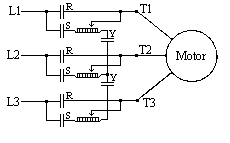Auto Transformer
Auto-Transformer Reduced Voltage

In this method, an auto-transformer is placed in series with the motor during staring. The transformer action reduces the voltage applied to the motor terminals. Because of the transformer action, the line current is less than the motor current. For a given starting current on the line side, the motor terminal voltage can be higher than for other reduced voltage start methods. Thus, this method gives the highest motor torque per line ampere.START: R OPEN, S & Y CLOSED
RUN: R CLOSED, S & Y OPEN

Auto-Transformer Reduced Voltage Starting

Starting Characteristics:

1. Motor terminal voltage less than line voltage (by transformer ratio).
2. Motor current exceeds line current (by inverse of transformer ratio).
3. Starting torque is reduced by the square of the terminal voltage.

Applications:

1. Where complete acceleration at reduced amperes is needed.
2. Where line ampere reduction requirements are severe, and load torque is not minimal.